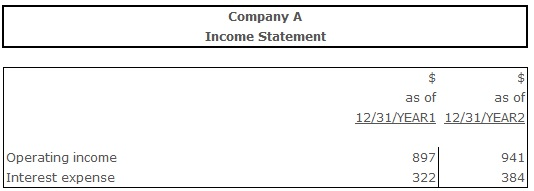# Times Interest Earned

Times Interest Earned (Interest Coverage Ratio) – an indicator of the firm’s debt-paying ability measuring the amount of income that can be used for covering interest expenses in future.

Involving debt to financing firm's activities necessarily means that operating earnings must be at least satisfying for making interest payments. Obviously, desirable are operating earnings that are much greater that the expenses that accompany the debt, which would indicate highly efficient use of the credit capital. Thus, the higher this ratio is, the better. If the times interest earned ratio is lower than 1, a firm most likely would not be able to meet its interest payments without issuing additional debt. 1 to 3 ranging values of this ratio can be a warning sign for a company, because this would mean that even though firm's debt-paying ability is on satisfactory level, it still can be easily affected by some minor changes, such as interest rates increase etc. Ratios ranging from 3 to 4 commonly are considered to be normal.

## Formula(s):

Times Interest Earned = (Recurring Earnings, Excluding Interest Expense, Tax Expense, Equity Earnings, and Noncontrolling Interest + Interest Expense) ÷ Interest Expense, Including Capitalized Interest

Times Interest Earned = EBIT ÷ Interest Expense

Times Interest Earned = Operating profit ÷ Interest expense

## Example:Times Interest Earned (Year 1) = 897 ÷ 322 = 2,78

Times Interest Earned (Year 2) = 941 ÷ 384 = 2,45

The times interest earned ratio indicates that the company had no problems generating enough cash flow to pay interest on its debt during the year 1-year 2 period. Company's ability to pay interest on debt slightly declined in year 2, but still remained on a very good level.

## Conclusion:

Times Interest Earned (also referred as Interest Coverage Ratio) indicates if a firm generates sufficient operating earnings for paying the interest expenses. Normative are the values ranging from 3 to 4, while ratios lower than 1 would mean that a firm is unable to meet its interest expenses. Positive for a firm is a stable and relatively high times interest earned ratio comparing to previous periods of its history. It would mean that the company is a reliable interest payer and so firm's management can expect obtaining funds for lower prices.